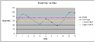# Finding upper and lower limits of a graph

Aki

## Homework Statement

This is not really a homework problem, but it looks like a rather easy problem that I can't quite figure out.

So I have a graph like shown in the attachment: a quantity vs time graph with some data points. I had no problem finding the average, but now I want to find both an upper and lower limits that would enclose 80% of the data points (so like what I have drawn in the graph). My question is: how can I do that using a simple program like Excel?

## Homework Equations

I tried applying Chebycheff's Inequality
http://en.wikipedia.org/wiki/Standard_deviation" [Broken]

For 80% of the data points, I found the number of standard deviations from the average would be SquareRoot 5. So (SquareRoot 5 * Standard Deviation)/2 , and then add and subtract that from the average to find the limits.

## The Attempt at a Solution

Chebycheff's Inequality worked for most graphs. However, for some graphs, I would get a negative value for the lower limit and that's not what I want to see.

Thanks for the help!

#### Attachments

•graph.JPG
22.5 KB · Views: 1,617
Last edited by a moderator: